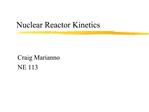DownloadDownload PresentationNuclear Reactor Kinetics

# Nuclear Reactor Kinetics

Download Presentation## Nuclear Reactor Kinetics

- - - - - - - - - - - - - - - - - - - - - - - - - - - E N D - - - - - - - - - - - - - - - - - - - - - - - - - - -
##### Presentation Transcript

1. Nuclear Reactor Kinetics Craig Marianno NE 113

2. Effective Multiplication Factor (keff)

3. keff and Power Power is directly proportional to neutron density keff = 1.0000 ? critical (power constant) keff < 1.0000 ? subcritical (power decreasing) keff > 1.0000 ? supercritical (power increasing)

4. k Excess Any difference between a given value for keff and 1.0000 is called the �excess� multiplication factor (?k) ?k = keff - 1.0000 = k excess

5. Reactivity When keff is close to 1.0000, ?k and ? and nearly the same. Example: keff = 0.98

6. Delayed Neutrons Single most important characteristic for reactor control Delayed neutrons ? decay of fission products (precursers) Prompt neutrons ? fission Fraction of delayed neutrons = ? Delayed neutrons are more effective than prompt because they are �born� at a somewhat lower energy.

7. Delayed Neutrons

8. Delayed Neutrons

9. Delayed Neutrons While it is true that they are only a small fraction of the total neutron population, they play a vital role in reactor kinetics. Why? They significantly increase the neutron cycle lifetime!

10. Prompt Critical

11. Prompt Critical

12. Reactivity in Dollars From our previous example:

13. Neutron Lifetime For reactor kinetics, it is important to know the average time elapsing between the release of a neutron in a fission reaction and its loss from the system either by absorption of escape. This is typically called the �prompt neutron lifetime�. This time can be divided into: 1) Slowing Down Time 2) Thermal Neutron Lifetime (Diffusion Time)

15. Neutron Lifetime (infinite medium - prompt only) ?a = total thermal macroscopic absorption cross section ?a = absorption mean free path v = mean velocity (2200 m s-1) Note: - finite size reduces average lifetime due to leakage - ?a for a core includes all materials

16. Effective Neutron Lifetime (delayed neutrons included) ?eff = effective fraction of delayed neutrons ?eff = effective decay constant of precursors ? = reactivity

17. Reactor Kinetics We need to construct an expression for the number of neutrons per second in the reactor during a given �neutron cycle�. We could use: n k l

18. Reactor Kinetics Solving:

19. Reactor Period To make the previous equation easier, we can define the reactor period (T) as T = l / ?k. The reactor period represents the length of time required to change the reactor power by a factor of e (2.718). This is why it is sometimes referred to as the �e folding time�.

20. Reactor Kinetics (Prompt Example) Assuming the following, what is the increase in power for a ?k = 0.0025 (\$0.357) at the end of 1.0 s? ?a = 13.2 cm v = 2200 m s-1

21. Reactor Kinetics (Delayed Example) Assuming the following, what is the increase in power for a ? = 0.0025 (\$0.357) at the end of 1.0 s? ? = 0.0813 s-1 ?eff = 0.007 v = 2200 m s-1 l = 6.0X10-5 s